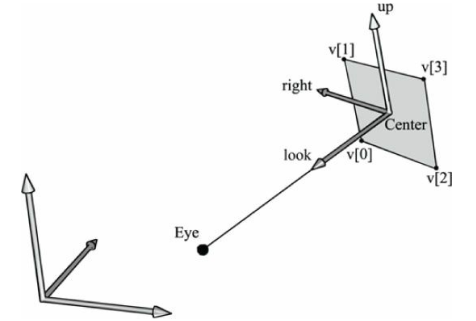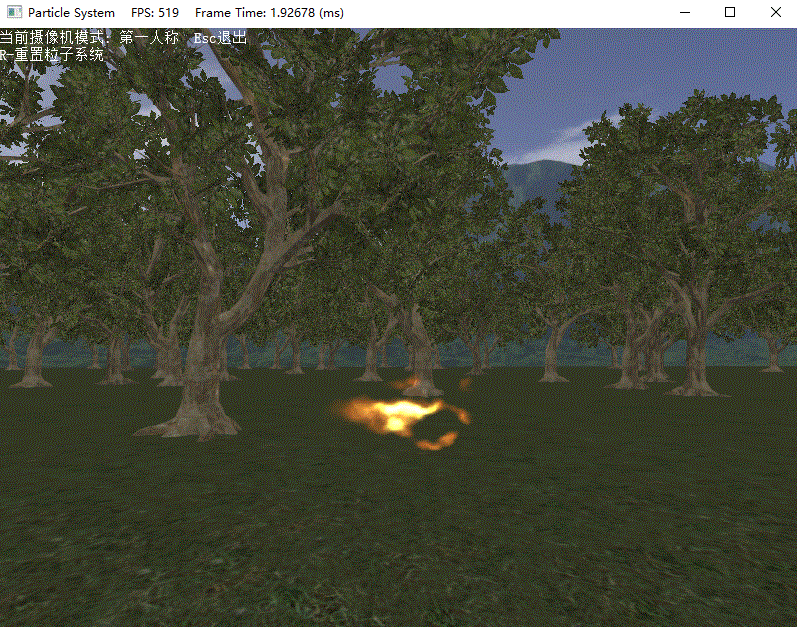# 前言

1. 熟悉如何利用几何着色器和流输出阶段来高效存储、渲染粒子
2. 了解我们如何利用基本的物理概念来让我们的粒子能够以物理上的真实方式来运动
3. 设计一个灵活的粒子系统框架使得我们可以方便地创建新的自定义粒子系统

DirectX11 With Windows SDK完整目录

Github项目源码

# 粒子的表示

w = E − C ∥ E − C ∥ u = j × w ∥ j × w ∥ v = w × u W = [ u x u y u z 0 v x v y v z 0 w x w y w z 0 C x C y C z 1 ] \mathbf{w}=\frac{\mathbf{E-C}}{\|\mathbf{E-C}\|}\\ \mathbf{u}=\frac{\mathbf{j\times w}}{\|\mathbf{j\times w}\|}\\ \mathbf{v}=\mathbf{w\times u}\\ \mathbf{W}=\begin{bmatrix} u_x & u_y & u_z & 0\\ v_x & v_y & v_z & 0\\ w_x & w_y & w_z & 0\\ C_x & C_y & C_z & 1\\ \end{bmatrix}struct Particle
{
XMFLOAT3 InitialPos;
XMFLOAT3 InitialVel;
XMFLOAT2 Size;
float Age;
unsigned int Type;
};


# 粒子运动

p(t)为粒子在t时刻的位置，它的运动轨迹为一条光滑曲线。它在t时刻的瞬时速度为：
v ( t ) = p ′ ( t ) \mathbf{v}(t)=\mathbf{p'}(t)

a ( t ) = v ′ ( t ) = p ′ ′ ( t ) \mathbf{a}(t)=\mathbf{v'}(t)=\mathbf{p''}(t)

∫ f ( t ) d t = F ( t ) + C [ F ( t ) + C ] ′ = f ( t ) \int\mathbf{f(t)}dt=\mathbf{F}(t) + C\\ [\mathbf{F}(t)+C]'=\mathbf{f}(t)

f(t)的不定积分得到的函数有无限个，即C可以为任意常数，这些函数求导后可以还原回f(t)

p ( t ) = ∫ v ( t ) d t v ( t ) = ∫ a ( t ) d t \mathbf{p}(t)=\int\mathbf{v}(t)dt\\ \mathbf{v}(t)=\int\mathbf{a}(t)dt\\

v ( t ) = ∫ a ( t ) d t = t ⋅ a + c \mathbf{v}(t)=\int\mathbf{a}(t)dt=t\cdot\mathbf{a} + \mathbf{c}

v ( 0 ) = c = v 0 \mathbf{v}(0)=\mathbf{c}=\mathbf{v_0}

v ( t ) = t ⋅ a + v 0 \mathbf{v}(t) =t\cdot\mathbf{a} + \mathbf{v_0}

p ( t ) = 1 2 t 2 a + t v 0 + p 0 \mathbf{p}(t) = \frac{1}{2}t^2\mathbf{a}+t\mathbf{v_0}+\mathbf{p_0}

# 随机性

float3 RandUnitVec3(float offset)
{
// 使用游戏时间加上偏移值来从随机纹理采样
float u = (g_GameTime + offset);
// 分量均在[-1,1]
float3 v = g_RandomVecMap.SampleLevel(g_SamLinear, u, 0).xyz;
// 标准化向量
return normalize(v);
}


# 混合与粒子系统

SrcBlend = SRC_ALPHA;
DestBlend = ONE;


C = a s ⋅ C s r c + C d s t \mathbf{C}=a_s\cdot\mathbf{C_{src}} + \mathbf{C_{dst}}

SrcBlend = ONE;
DestBlend = ONE;


# 基于GPU的粒子系统

## 粒子系统特效

1. 摧毁条件不同：我们可能要在雨水打中地面的时候将它摧毁，然而对于火焰粒子来说它是在几秒钟后被摧毁
2. 变化过程不同：烟雾粒子可能随着时间推移变得暗淡，对于雨水粒子来说并不是这样的。同样地，烟雾粒子随时间推移逐渐扩散，而雨水大多是往下掉落的。
3. 绘制过程不同：线图元通常在模拟雨水的时候效果良好，但火焰/烟雾粒子更多使用的是公告板的四边形。
4. 生成条件不同：雨水和烟雾的初始位置和速度的设置方式明显也是不同的。

## ParticleEffect类

class ParticleEffect : public IEffect
{
public:
ParticleEffect();
virtual ~ParticleEffect() override;

ParticleEffect(ParticleEffect&& moveFrom) noexcept;
ParticleEffect& operator=(ParticleEffect&& moveFrom) noexcept;

// 初始化所需资源
// 若effectPath为HLSL/Fire
// 则会寻找文件:
// - HLSL/Fire_SO_VS.hlsl
// - HLSL/Fire_SO_GS.hlsl
// - HLSL/Fire_VS.hlsl
// - HLSL/Fire_GS.hlsl
// - HLSL/Fire_PS.hlsl
bool Init(ID3D11Device* device, const std::wstring& effectPath);

// 产生新粒子到顶点缓冲区
void SetRenderToVertexBuffer(ID3D11DeviceContext* deviceContext);
// 绘制粒子系统
void SetRenderDefault(ID3D11DeviceContext* deviceContext);

void XM_CALLCONV SetViewProjMatrix(DirectX::FXMMATRIX VP);

void SetEyePos(const DirectX::XMFLOAT3& eyePos);

void SetGameTime(float t);
void SetTimeStep(float step);

void SetEmitDir(const DirectX::XMFLOAT3& dir);
void SetEmitPos(const DirectX::XMFLOAT3& pos);

void SetEmitInterval(float t);
void SetAliveTime(float t);

void SetBlendState(ID3D11BlendState* blendState, const FLOAT blendFactor, UINT sampleMask);
void SetDepthStencilState(ID3D11DepthStencilState* depthStencilState, UINT stencilRef);

void SetDebugObjectName(const std::string& name);

//
// IEffect
//

// 应用常量缓冲区和纹理资源的变更
void Apply(ID3D11DeviceContext* deviceContext) override;

private:
class Impl;
std::unique_ptr<Impl> pImpl;
};


## ParticleRender类

class ParticleRender
{
public:
template<class T>
using ComPtr = Microsoft::WRL::ComPtr<T>;

ParticleRender() = default;
~ParticleRender() = default;
// 不允许拷贝，允许移动
ParticleRender(const ParticleRender&) = delete;
ParticleRender& operator=(const ParticleRender&) = delete;
ParticleRender(ParticleRender&&) = default;
ParticleRender& operator=(ParticleRender&&) = default;

// 自从该系统被重置以来所经过的时间
float GetAge() const;

void SetEmitPos(const DirectX::XMFLOAT3& emitPos);
void SetEmitDir(const DirectX::XMFLOAT3& emitDir);

void SetEmitInterval(float t);
void SetAliveTime(float t);

HRESULT Init(ID3D11Device* device, UINT maxParticles);

void Reset();
void Update(float dt, float gameTime);
void Draw(ID3D11DeviceContext* deviceContext, ParticleEffect& effect, const Camera& camera);

void SetDebugObjectName(const std::string& name);

private:

UINT m_MaxParticles = 0;
bool m_FirstRun = true;

float m_GameTime = 0.0f;
float m_TimeStep = 0.0f;
float m_Age = 0.0f;

DirectX::XMFLOAT3 m_EmitPos = {};
DirectX::XMFLOAT3 m_EmitDir = {};

float m_EmitInterval = 0.0f;
float m_AliveTime = 0.0f;

ComPtr<ID3D11Buffer> m_pInitVB;
ComPtr<ID3D11Buffer> m_pDrawVB;
ComPtr<ID3D11Buffer> m_pStreamOutVB;

};


**注意：**粒子系统使用一个纹理数组来对粒子进行贴图，因为我们可能不想让所有的粒子看起来都是一样的。例如，为了实现一个烟雾的粒子系统，我们可能想要使用几种烟雾纹理来添加变化，图元ID在像素着色器中可以用来对纹理数组进行索引。

## 起始顶点缓冲区

void ParticleRender::Reset()
{
m_FirstRun = true;
m_Age = 0.0f;
}


## 更新/绘制过程

1. 通过流输出几何着色器阶段来更新当前帧的粒子
2. 使用更新好的粒子进行渲染
void ParticleRender::Draw(ID3D11DeviceContext* deviceContext, ParticleEffect& effect, const Camera& camera)
{
effect.SetGameTime(m_GameTime);
effect.SetTimeStep(m_TimeStep);
effect.SetEmitPos(m_EmitPos);
effect.SetEmitDir(m_EmitDir);
effect.SetEmitInterval(m_EmitInterval);
effect.SetAliveTime(m_AliveTime);
effect.SetTextureArray(m_pTextureArraySRV.Get());
effect.SetTextureRandom(m_pRandomTexSRV.Get());

// ******************
// 流输出
//
effect.SetRenderToVertexBuffer(deviceContext);
UINT strides = { sizeof(VertexParticle) };
UINT offsets = { 0 };

// 如果是第一次运行，使用初始顶点缓冲区
// 否则，使用存有当前所有粒子的顶点缓冲区
if (m_FirstRun)
else

// 经过流输出写入到顶点缓冲区
effect.Apply(deviceContext);
if (m_FirstRun)
{
deviceContext->Draw(1, 0);
m_FirstRun = false;
}
else
{
deviceContext->DrawAuto();
}

// 解除缓冲区绑定
ID3D11Buffer* nullBuffers = { nullptr };
deviceContext->SOSetTargets(1, nullBuffers, offsets);

// 进行顶点缓冲区的Ping-Pong交换
m_pDrawVB.Swap(m_pStreamOutVB);

// ******************
// 使用流输出顶点绘制粒子
//
effect.SetRenderDefault(deviceContext);

effect.Apply(deviceContext);
deviceContext->DrawAuto();
}


# 火焰

// Fire.hlsli

cbuffer CBChangesEveryFrame : register(b0)
{
matrix g_ViewProj;

float3 g_EyePosW;
float g_GameTime;

float g_TimeStep;
float3 g_EmitDirW;

float3 g_EmitPosW;
float g_EmitInterval;

float g_AliveTime;
}

cbuffer CBFixed : register(b1)
{
// 用于加速粒子运动的加速度
float3 g_AccelW = float3(0.0f, 7.8f, 0.0f);

// 纹理坐标
{
float2(0.0f, 1.0f),
float2(1.0f, 1.0f),
float2(0.0f, 0.0f),
float2(1.0f, 0.0f)
};
}

// 用于贴图到粒子上的纹理数组
Texture2DArray g_TexArray : register(t0);

// 用于在着色器中生成随机数的纹理
Texture1D g_RandomTex : register(t1);

// 采样器
SamplerState g_SamLinear : register(s0);

float3 RandUnitVec3(float offset)
{
// 使用游戏时间加上偏移值来采样随机纹理
float u = (g_GameTime + offset);

// 采样值在[-1,1]
float3 v = g_RandomTex.SampleLevel(g_SamLinear, u, 0).xyz;

// 投影到单位球
return normalize(v);
}

#define PT_EMITTER 0
#define PT_FLARE 1

struct VertexParticle
{
float3 InitialPosW : POSITION;
float3 InitialVelW : VELOCITY;
float2 SizeW : SIZE;
float Age : AGE;
uint Type : TYPE;
};

// 绘制输出
struct VertexOut
{
float3 PosW : POSITION;
float2 SizeW : SIZE;
float4 Color : COLOR;
uint Type : TYPE;
};

struct GeoOut
{
float4 PosH : SV_Position;
float4 Color : COLOR;
float2 Tex : TEXCOORD;
};


// Fire_SO_VS.hlsl
#include "Fire.hlsli"

VertexParticle VS(VertexParticle vIn)
{
return vIn;
}


// Fire_SO_GS.hlsl
#include "Fire.hlsli"

[maxvertexcount(2)]
void GS(point VertexParticle gIn, inout PointStream<VertexParticle> output)
{
gIn.Age += g_TimeStep;

if (gIn.Type == PT_EMITTER)
{
// 是否到时间发射新的粒子
if (gIn.Age > g_EmitInterval)
{
float3 vRandom = RandUnitVec3(0.0f);
vRandom.x *= 0.5f;
vRandom.z *= 0.5f;

VertexParticle p;
p.InitialPosW = g_EmitPosW.xyz;
p.InitialVelW = 4.0f * vRandom;
p.SizeW       = float2(3.0f, 3.0f);
p.Age         = 0.0f;
p.Type = PT_FLARE;

output.Append(p);

// 重置时间准备下一次发射
gIn.Age = 0.0f;
}

// 总是保留发射器
output.Append(gIn);
}
else
{
// 用于限制粒子数目产生的特定条件，对于不同的粒子系统限制也有所变化
if (gIn.Age <= g_AliveTime)
output.Append(gIn);
}
}


// Fire_VS.hlsl
#include "Fire.hlsli"

VertexOut VS(VertexParticle vIn)
{
VertexOut vOut;

float t = vIn.Age;

// 恒定加速度等式
vOut.PosW = 0.5f * t * t * g_AccelW + t * vIn.InitialVelW + vIn.InitialPosW;

// 颜色随着时间褪去
float opacity = 1.0f - smoothstep(0.0f, 1.0f, t / 1.0f);
vOut.Color = float4(1.0f, 1.0f, 1.0f, opacity);

vOut.SizeW = vIn.SizeW;
vOut.Type = vIn.Type;

return vOut;
}


// Fire_GS.hlsl
#include "Fire.hlsli"

[maxvertexcount(4)]
void GS(point VertexOut gIn, inout TriangleStream<GeoOut> output)
{
// 不要绘制用于产生粒子的顶点
if (gIn.Type != PT_EMITTER)
{
//
// 计算该粒子的世界矩阵让公告板朝向摄像机
//
float3 look  = normalize(g_EyePosW.xyz - gIn.PosW);
float3 right = normalize(cross(float3(0.0f, 1.0f, 0.0f), look));
float3 up = cross(look, right);

//
// 计算出处于世界空间的四边形
//
float halfWidth  = 0.5f * gIn.SizeW.x;
float halfHeight = 0.5f * gIn.SizeW.y;

float4 v;
v = float4(gIn.PosW + halfWidth * right - halfHeight * up, 1.0f);
v = float4(gIn.PosW + halfWidth * right + halfHeight * up, 1.0f);
v = float4(gIn.PosW - halfWidth * right - halfHeight * up, 1.0f);
v = float4(gIn.PosW - halfWidth * right + halfHeight * up, 1.0f);

//
// 将四边形顶点从世界空间变换到齐次裁减空间
//
GeoOut gOut;
[unroll]
for (int i = 0; i < 4; ++i)
{
gOut.PosH  = mul(v[i], g_ViewProj);
gOut.Color = gIn.Color;
output.Append(gOut);
}
}
}


// Fire_PS.hlsl
#include "Fire.hlsli"

float4 PS(GeoOut pIn) : SV_Target
{
return g_TexArray.Sample(g_SamLinear, float3(pIn.Tex, 0.0f)) * pIn.Color;
}



m_pFireEffect->SetBlendState(RenderStates::BSAlphaWeightedAdditive.Get(), nullptr, 0xFFFFFFFF);
m_pFireEffect->SetDepthStencilState(RenderStates::DSSNoDepthWrite.Get(), 0);


# 雨水

// Rain.hlsli

cbuffer CBChangesEveryFrame : register(b0)
{
matrix g_ViewProj;

float3 g_EyePosW;
float g_GameTime;

float g_TimeStep;
float3 g_EmitDirW;

float3 g_EmitPosW;
float g_EmitInterval;

float g_AliveTime;
}

cbuffer CBFixed : register(b1)
{
// 用于加速粒子运动的加速度
float3 g_AccelW = float3(-1.0f, -9.8f, 0.0f);
}

// 用于贴图到粒子上的纹理数组
Texture2DArray g_TexArray : register(t0);

// 用于在着色器中生成随机数的纹理
Texture1D g_RandomTex : register(t1);

// 采样器
SamplerState g_SamLinear : register(s0);

float3 RandUnitVec3(float offset)
{
// 使用游戏时间加上偏移值来采样随机纹理
float u = (g_GameTime + offset);

// 采样值在[-1,1]
float3 v = g_RandomTex.SampleLevel(g_SamLinear, u, 0).xyz;

// 投影到单位球
return normalize(v);
}

float3 RandVec3(float offset)
{
// 使用游戏时间加上偏移值来采样随机纹理
float u = (g_GameTime + offset);

// 采样值在[-1,1]
float3 v = g_RandomTex.SampleLevel(g_SamLinear, u, 0).xyz;

return v;
}

#define PT_EMITTER 0
#define PT_FLARE 1

struct VertexParticle
{
float3 InitialPosW : POSITION;
float3 InitialVelW : VELOCITY;
float2 SizeW       : SIZE;
float Age          : AGE;
uint Type         : TYPE;
};

// 绘制输出
struct VertexOut
{
float3 PosW : POSITION;
uint Type : TYPE;
};

struct GeoOut
{
float4 PosH : SV_Position;
float2 Tex : TEXCOORD;
};


// Rain_SO_VS.hlsl
#include "Rain.hlsli"

VertexParticle VS(VertexParticle vIn)
{
return vIn;
}


// Rain_SO_GS.hlsl
#include "Rain.hlsli"

[maxvertexcount(6)]
void GS(point VertexParticle gIn, inout PointStream<VertexParticle> output)
{
gIn.Age += g_TimeStep;

if (gIn.Type == PT_EMITTER)
{
// 是否到时间发射新的粒子
if (gIn.Age > g_EmitInterval)
{
[unroll]
for (int i = 0; i < 5; ++i)
{
// 在摄像机上方的区域让雨滴降落
float3 vRandom = 30.0f * RandVec3((float)i / 5.0f);
vRandom.y = 20.0f;

VertexParticle p;
p.InitialPosW = g_EmitPosW.xyz + vRandom;
p.InitialVelW = float3(0.0f, 0.0f, 0.0f);
p.SizeW       = float2(1.0f, 1.0f);
p.Age         = 0.0f;
p.Type        = PT_FLARE;

output.Append(p);
}

// 重置时间准备下一次发射
gIn.Age = 0.0f;
}

// 总是保留发射器
output.Append(gIn);
}
else
{
// 用于限制粒子数目产生的特定条件，对于不同的粒子系统限制也有所变化
if (gIn.Age <= g_AliveTime)
output.Append(gIn);
}
}


// Rain_VS.hlsl
#include "Rain.hlsli"

VertexOut VS(VertexParticle vIn)
{
VertexOut vOut;

float t = vIn.Age;

// 恒定加速度等式
vOut.PosW = 0.5f * t * t * g_AccelW + t * vIn.InitialVelW + vIn.InitialPosW;

vOut.Type = vIn.Type;

return vOut;
}


// Rain_GS.hlsl
#include "Rain.hlsli"

[maxvertexcount(6)]
void GS(point VertexOut gIn, inout LineStream<GeoOut> output)
{
// 不要绘制用于产生粒子的顶点
if (gIn.Type != PT_EMITTER)
{
// 使线段沿着一个加速度方向倾斜
float3 p0 = gIn.PosW;
float3 p1 = gIn.PosW + 0.07f * g_AccelW;

GeoOut v0;
v0.PosH = mul(float4(p0, 1.0f), g_ViewProj);
v0.Tex = float2(0.0f, 0.0f);
output.Append(v0);

GeoOut v1;
v1.PosH = mul(float4(p1, 1.0f), g_ViewProj);
v1.Tex = float2(0.0f, 0.0f);
output.Append(v1);
}
}


// Rain_PS.hlsl
#include "Rain.hlsli"

float4 PS(GeoOut pIn) : SV_Target
{
return g_TexArray.Sample(g_SamLinear, float3(pIn.Tex, 0.0f));
}



m_pRainEffect->SetDepthStencilState(RenderStates::DSSNoDepthWrite.Get(), 0);


# C++代码实现

bool GameApp::InitResource()
{
// ...

// ******************
// 初始化特效
//

// ...

m_pFireEffect->SetDepthStencilState(RenderStates::DSSNoDepthWrite.Get(), 0);

m_pRainEffect->SetDepthStencilState(RenderStates::DSSNoDepthWrite.Get(), 0);

// ...

// ******************
// 初始化粒子系统
//
HR(CreateTexture2DArrayFromFile(m_pd3dDevice.Get(), m_pd3dImmediateContext.Get(),
m_pFire->Init(m_pd3dDevice.Get(), 500);
m_pFire->SetTextureArraySRV(pFlareSRV.Get());
m_pFire->SetRandomTexSRV(pRandomSRV.Get());
m_pFire->SetEmitPos(XMFLOAT3(0.0f, -1.0f, 0.0f));
m_pFire->SetEmitDir(XMFLOAT3(0.0f, 1.0f, 0.0f));
m_pFire->SetEmitInterval(0.005f);
m_pFire->SetAliveTime(1.0f);

HR(CreateTexture2DArrayFromFile(m_pd3dDevice.Get(), m_pd3dImmediateContext.Get(),
m_pRain->Init(m_pd3dDevice.Get(), 10000);
m_pRain->SetTextureArraySRV(pRainSRV.Get());
m_pRain->SetRandomTexSRV(pRandomSRV.Get());
m_pRain->SetEmitDir(XMFLOAT3(0.0f, -1.0f, 0.0f));
m_pRain->SetEmitInterval(0.0015f);
m_pRain->SetAliveTime(3.0f);

// ...
}


void GameApp::UpdateScene(float dt)
{
// ...

// ******************
// 粒子系统
//
if (m_KeyboardTracker.IsKeyPressed(Keyboard::R))
{
m_pFire->Reset();
m_pRain->Reset();
}
m_pFire->Update(dt, m_Timer.TotalTime());
m_pRain->Update(dt, m_Timer.TotalTime());

m_pFireEffect->SetViewProjMatrix(m_pCamera->GetViewProjXM());
m_pFireEffect->SetEyePos(m_pCamera->GetPosition());

static XMFLOAT3 lastCameraPos = m_pCamera->GetPosition();
XMFLOAT3 cameraPos = m_pCamera->GetPosition();

XMFLOAT3 emitPos;
XMStoreFloat3(&emitPos, cameraPosVec + 3.0f * (cameraPosVec - lastCameraPosVec));
m_pRainEffect->SetViewProjMatrix(m_pCamera->GetViewProjXM());
m_pRainEffect->SetEyePos(m_pCamera->GetPosition());
m_pRain->SetEmitPos(emitPos);
lastCameraPos = m_pCamera->GetPosition();
}


void GameApp::DrawScene()
{
assert(m_pd3dImmediateContext);
assert(m_pSwapChain);

m_pd3dImmediateContext->ClearRenderTargetView(m_pRenderTargetView.Get(), reinterpret_cast<const float*>(&Colors::Silver));
m_pd3dImmediateContext->ClearDepthStencilView(m_pDepthStencilView.Get(), D3D11_CLEAR_DEPTH | D3D11_CLEAR_STENCIL, 1.0f, 0);

// ******************
// 正常绘制场景
//

// 统计实际绘制的物体数目
std::vector<Transform> acceptedData;
// 默认视锥体裁剪
acceptedData = Collision::FrustumCulling(m_InstancedData, m_Trees.GetLocalBoundingBox(),
m_pCamera->GetViewXM(), m_pCamera->GetProjXM());
// 默认硬件实例化绘制
m_pBasicEffect->SetRenderDefault(m_pd3dImmediateContext.Get(), BasicEffect::RenderInstance);
m_Trees.DrawInstanced(m_pd3dImmediateContext.Get(), m_pBasicEffect.get(), acceptedData);

// 绘制地面
m_pBasicEffect->SetRenderDefault(m_pd3dImmediateContext.Get(), BasicEffect::RenderObject);
m_Ground.Draw(m_pd3dImmediateContext.Get(), m_pBasicEffect.get());

// 绘制天空盒
m_pSkyEffect->SetRenderDefault(m_pd3dImmediateContext.Get());
m_pGrassCube->Draw(m_pd3dImmediateContext.Get(), *m_pSkyEffect, *m_pCamera);

// ******************
// 粒子系统留在最后绘制
//

m_pFire->Draw(m_pd3dImmediateContext.Get(), *m_pFireEffect, *m_pCamera);
m_pRain->Draw(m_pd3dImmediateContext.Get(), *m_pRainEffect, *m_pCamera);

// ...
}


# 演示# 练习题

1. 实现一个爆炸的粒子系统。发射器粒子产生N个随机方向的外壳粒子。在经过一个短暂时间后，每个外壳粒子应当爆炸产生M个粒子。每个外壳不需要在同一个时间发生爆炸——通过随机性赋上不同的爆炸倒计时。对M和N进行测试直到你得到不错的结果。注意发射器在产生所有的外壳粒子后，将其摧毁使得不要产生更多的外壳。
2. 实现一个喷泉的粒子系统。这些粒子应当从某个点产生，并沿着圆锥体范围内的随机方向向上发射。最终重力会使得它们掉落到地面。注意：给粒子一个足够高的初速度来让它们克服重力。

DirectX11 With Windows SDK完整目录

Github项目源码

09-132万+
12-29561908-2988
03-232万+
08-0164
06-175815
06-192742
07-191万+